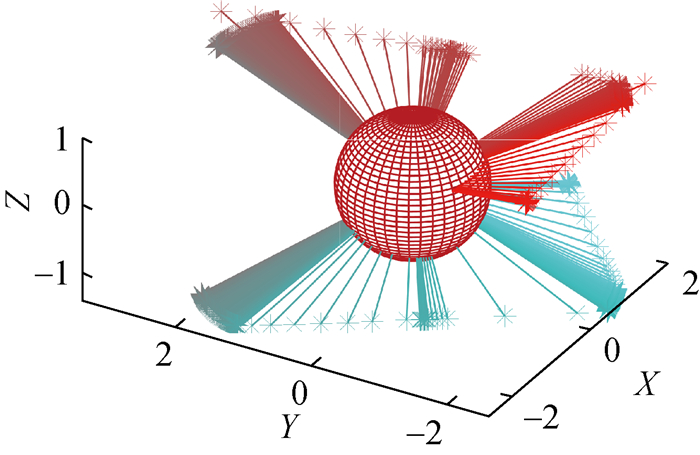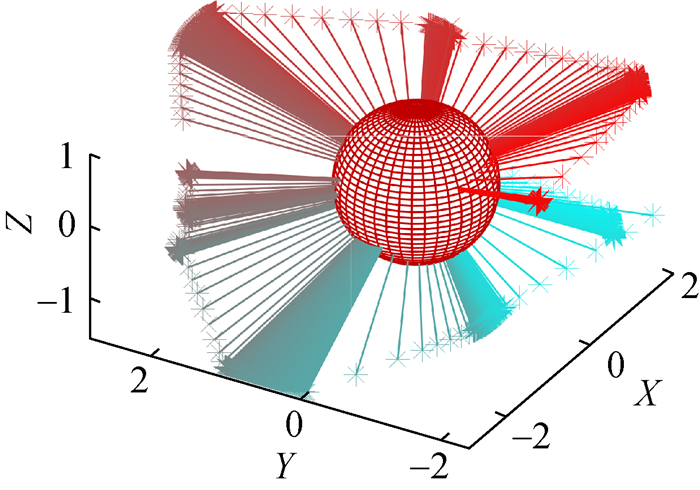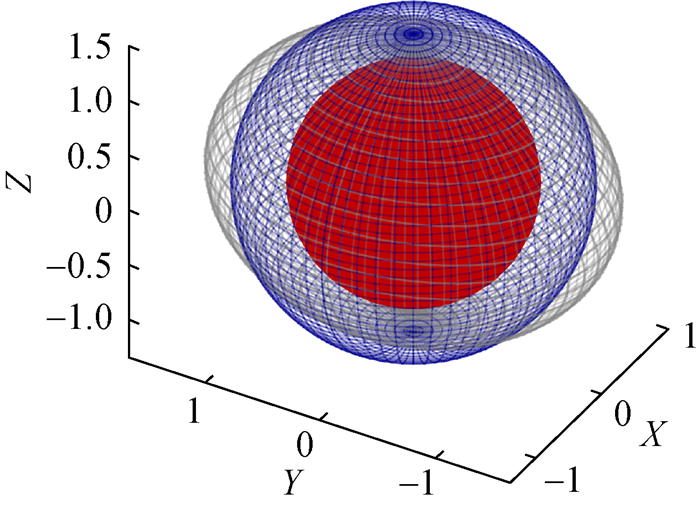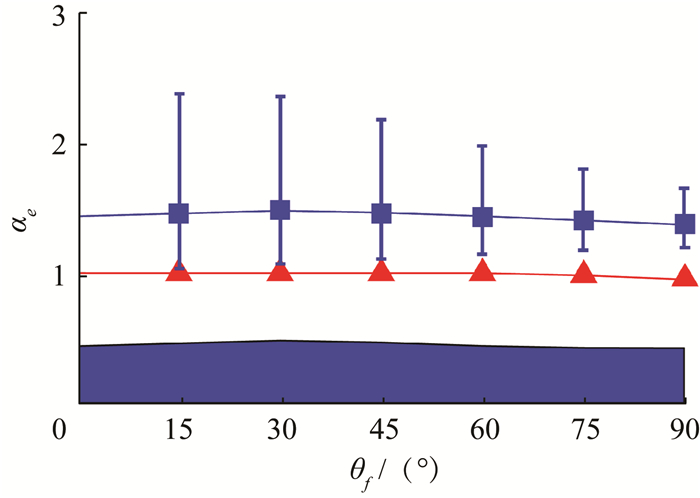Assessing spacecraft agility
YIN Mingwei, WANG Xianyu, LI Jingyang,
School of Aerospace Engineering, Tsinghua University, Beijing 10084, China
Abstract: When increasing the spacecraft agility by optimal control, risk and benefits coexist. A method was developed to assess spacecraft agility and estimate the agility enhancement. The model analyzes the conventional eigenaxis rotation and the time-optimal rotation. Then, the reorientation time was used to define an angular acceleration envelope and an equivalent agility envelope with an agility factor and an agility curve introduced to quantitatively assess the spacecraft agility. Numerical simulations validate the reliabilities of the estimates. The results are consistent with previous research and the average reorientation time is well predicted. Thus, this method is suitable for assessing spacecraft agility.
Key words: agile satellite     Euler rotation     time optimal     maneuver capability     agility factor

1 航天器传统姿态机动 1.1 航天器模型图 1 航天器姿态机动模型

 $\left\{ \begin{array}{l} {I_1}{{\dot \omega }_1} - ({I_2} - {I_3}){\omega _2}{\omega _3} = {u_1}, \\ {I_2}{{\dot \omega }_2} - ({I_3} - {I_1}){\omega _3}{\omega _1} = {u_2}, {\rm{ }}\\ {I_3}{{\dot \omega }_3} - ({I_1} - {I_2}){\omega _1}{\omega _2} = {u_3}. \end{array} \right.$ (1)

 $\left\{ \begin{array}{l} {I_1} + {I_2} \ge {I_3}, \\ {I_1} + {I_3} \ge {I_2}, {\rm{ }}\\ {I_2} + {I_3} \ge {I_1}. \end{array} \right.$ (2)

 $\left| {{u_i}} \right| \le {u_{{\rm{max}}}}, {\rm{ }}i = 1, {\rm{ }}2, {\rm{ }}3.$ (3)

 $\begin{array}{l} \dot q = \frac{1}{2}\left[ \begin{array}{l} \;\;\;0\;\;\;\;\;\;\;{\omega _3}\;\;\; - {\omega _2}\;{\omega _1}\\ - {\omega _3}\;\;\;\;\;\;0\;\;\;\;\;\;\;{\omega _1}\;\;{\omega _2}\\ \;\;{\omega _2}\;\;\;- {\omega _1}\;\;\;\;\;\;0\;\;\;{\omega _3}\\ - {\omega _1}\;\; - {\omega _2}\;\; - {\omega _3}\;\;0 \end{array} \right]\left[ \begin{array}{l} {q_1}\\ {q_2}\\ {q_3}\\ {q_4} \end{array} \right] = \\ \;\;\;\;\;\;\;\;\;\;\;\;\;\;\;\;\;\;\;\;\;\;\;\;\frac{1}{2}\mathit{\pmb{\Omega q}}. \end{array}$ (4)

q的4个分量满足关系式

 $q_1^2 + q_2^2 + q_3^2 + q_4^2 = 1.$ (5)

1.2 传统姿态机动

 $\alpha = \frac{u}{I}.$ (6)

 ${I_{{\rm{max}}}} = {\rm{max}}\left\{ {{I_1}, {I_2}, {I_3}} \right\}.$ (7)

 ${\alpha _{{\rm{max}}}} = \frac{{{u_{{\rm{max}}}}}}{{{I_{{\rm{max}}}}}}.$ (8)

 $\left| {{\omega _i}} \right| \le {\omega _{{\rm{max}}}}, {\rm{ }}i = 1, 2, 3.$ (9)

 ${t_f} = \sqrt {\frac{{4{\theta _f}}}{{{\alpha _{{\rm{max}}}}}}} .$ (10)

 ${t_{{\rm{up}}}} = \frac{{{\omega _{{\rm{max}}}}}}{{{\alpha _{{\rm{max}}}}}}.$ (11)

 ${t_c} = \frac{{{\theta _f} - {\theta _{{\rm{cri}}}}}}{{{\omega _{{\rm{max}}}}}},$ (12)

 ${\theta _{{\rm{cri}}}} = \frac{{\omega _{{\rm{max}}}^2}}{{{\alpha _{{\rm{max}}}}}}.$ (13)

θf < θcri时，机动过程中角速度总是小于ωmax，Euler旋转的时间由式(10)可求得；而θf>θcri时，则需考虑最大角速度的限制，Euler旋转的时间为

 ${t_f} = 2{t_{{\rm{up}}}} + {t_{\rm{c}}} = \frac{{{\omega _{{\rm{max}}}}}}{{{\alpha _{{\rm{max}}}}}} + \frac{{{\theta _f}}}{{{\omega _{{\rm{max}}}}}}.$ (14)
2 时间最优姿态机动 2.1 优化问题模型

 $\begin{array}{l} \;\;\;\;\;\;\;\;{\rm{min}}J = {t_f}, \\ \begin{array}{*{20}{l}} {{\rm{s}}{\rm{.t}}{\rm{.}}}\\ {}\\ {}\\ {}\\ {}\\ {}\\ {}\\ \begin{array}{l} \\ \end{array} \end{array}\left\{ \begin{array}{l} {I_1}{{\dot \omega }_1} - ({I_2} - {I_3}){\omega _2}{\omega _3} = {u_1}, \\ {I_2}{{\dot \omega }_2} - ({I_3} - {I_1}){\omega _3}{\omega _1} = {u_2}, \\ {I_3}{{\dot \omega }_3} - ({I_1} - {I_2}){\omega _1}{\omega _2} = {u_3}, \\ \dot q = \frac{1}{2}\mathit{\pmb{\Omega q}}, \\ \left| {{u_i}} \right| \le {u_{{\rm{max}}}}, {\rm{ }}i = 1, 2, 3, \\ \left| {{\omega _i}} \right| \le {\omega _{{\rm{max}}}}, {\rm{ }}i = 1, 2, 3, \\ {\mathit{\pmb{q}}_0}, {\rm{ }}{\mathit{\pmb{q}}_f}, \\ {\mathit{\pmb\omega{}} _0} = {\mathit{\pmb\omega{}} _f} = 0. \end{array} \right. \end{array}$ (15)

2.2 时间最优姿态机动

 主要参数 参数值 I1 0.809 I2 0.421 I3 1 umax 1 ωmax 1图 2 (网络版彩图)绕Z轴变化45°时最优机动的瞬时角加速度图 3 (网络版彩图)绕Z轴变化90°时最优机动的瞬时角加速度

3 敏捷性评估 3.1 敏捷因子

Mark等认为提高敏捷性就是缩短航天器完成姿态机动所需的时间，Wie认为影响敏捷卫星性能的主要因素就是平均再定位时间，在航空领域，为了评估战斗机的横滚敏捷性，也是根据战斗机横滚90°所需要的时间来评价。这些观点里面都提及到了时间，完成机动所需的时间越短，则航天器的敏捷性越好，这与对敏捷性的直观感受不谋而合。而且时间作为结果，可以将机动过程中的所有约束条件均考虑进来。此外，需要注意的是，航天器完成不同角度的机动时，由于受到限制条件的不同，其敏捷性是不一样的。综上，可以用航天器完成特定姿态机动所需的时间作为中间量，构造等效角加速度，从而评估航天器姿态机动的敏捷性。

 ${\alpha _X} = \frac{{4{\theta _f}}}{{t_X^2}}.$ (16)

 ${\alpha _Y} = \frac{{4{\theta _f}}}{{t_Y^2}}，$ (17)
 ${\alpha _Z} = \frac{{4{\theta _f}}}{{t_Z^2}}.$ (18)

 ${\alpha _e} = 4{\theta _f}{({t_X}{t_Y}{t_Z})^{ - \frac{2}{3}}}.$ (19)图 4 (网络版彩图)航天器XTE机动90°的等效敏捷包络

3.2 敏捷性曲线图 5 (网络版彩图)航天器XTE机动0°~90°的敏捷性曲线

4 仿真算例

4.1 对比分析

4.2 预估机动时间图 6 (网络版彩图)航天器XTE绕不同方向机动45°的时间包络

5 结论

  WIE B, BAILEY D, HEIBERG C. Rapid multitarget acquisition and pointing control of agile spacecraft[J]. Journal of Guidance, Control, and Dynamics, 2002, 25(1): 96-104.  靳瑾, 张景瑞, 刘藻珍. 航天器大角度姿态快速机动控制器参数优化设计[J]. 清华大学学报(自然科学版), 2009(2): 289-292. JIN J, ZHANG J R, LIU Z Z. Optimized design of controller parameters for large angle spacecraft attitude maneuvers[J]. Journal of Tsinghua University (Science and Technology), 2009(2): 289-292. DOI:10.3321/j.issn:1000-0054.2009.02.033 (in Chinese)  LEMAÎTRE M, VERFAILLIE G, JOUHAUD F, et al. Selecting and scheduling observations of agile satellites[J]. Aerospace Science and Technology, 2002, 6(5): 367-381. DOI:10.1016/S1270-9638(02)01173-2  CAO X, YUE C, LIU M, et al. Time efficient spacecraft maneuver using constrained torque distribution[J]. Acta Astronautica, 2016, 123: 320-329. DOI:10.1016/j.actaastro.2016.03.026  印明威, 李京阳, 宝音贺西. 敏捷卫星姿态机动的奇异最优控制[J]. 光学精密工程, 2018, 26(4): 906-915. YIN M W, LI J Y, BAOYIN H X. Singular optimal control for three-axis reorientation of an agile satellite[J]. Optics and Precision Engineering, 2018, 26(4): 906-915. (in Chinese)  YIN M, LI J, WANG X, et al. A rapid method for validation and visualization of agile earth-observation satellites scheduling[J]. Astrodynamics, 2018, 2(4): 325-337. DOI:10.1007/s42064-018-0026-9  KARPENKO M, PROULX R J. Experimental implementation of Riemann—Stieltjes optimal control for agile imaging satellites[J]. Journal of Guidance, Control, and Dynamics, 2016, 39(1): 144-150.  KING J T, KARPENKO M. A simple approach for predicting time-optimal slew capability[J]. Acta Astronautica, 2016, 120: 159-170. DOI:10.1016/j.actaastro.2015.12.009  KARPENKO M, BHATT S, BEDROSSIAN N, et al. First flight results on time-optimal spacecraft slews[J]. Journal of Guidance, Control, and Dynamics, 2012, 35(2): 367-376.  KARPENKO M, BHATT S, BEDROSSIAN N, et al. Flight implementation of shortest-time maneuvers for imaging satellites[J]. Journal of Guidance, Control, and Dynamics, 2014, 37(4): 1069-1079.  曾祥远, 龚胜平, 高云峰, 等. 非理想太阳帆航天器时间最优交会任务[J]. 清华大学学报(自然科学版), 2014, 54(9): 1240-1244, 1254. ZENG X Y, GONG S P, GAO Y F, et al. Non-ideal solar sailcraft time-optimal rendezvous mission[J]. Journal of Tsinghua University (Science and Technology), 2014, 54(9): 1240-1244, 1254. (in Chinese)  徐利民, 张涛. 基于外扰观测器的双通道航天器姿态控制方法[J]. 清华大学学报(自然科学版), 2017, 57(6): 631-636. XU L M, ZHANG T. Dual channel spacecraft attitude control method based on an external disturbance observer[J]. Journal of Tsinghua University (Science and Technology), 2017, 57(6): 631-636. (in Chinese)  程磊, 王天舒, 李俊峰. 挠性多体卫星姿态动力学与控制[J]. 清华大学学报(自然科学版), 2005, 45(11): 1506-1509. CHENG L, WANG T S, LI J F. Attitude dynamics and control of a flexible multi-body satellite[J]. Journal of Tsinghua University (Science and Technology), 2005, 45(11): 1506-1509. DOI:10.3321/j.issn:1000-0054.2005.11.017 (in Chinese)  YANG H W, LI S, BAI X L. Fast homotopy method for asteroid landing trajectory optimization using approximate initial co-states[J]. Journal of Guidance, Control, and Dynamics, 2019, 42(3): 585-597.  ROSS I M, KARPENKO M. A review of pseudospectral optimal control: From theory to flight[J]. Annual Reviews in Control, 2012, 36(2): 182-197. DOI:10.1016/j.arcontrol.2012.09.002  KARPENKO M, KING J T, DENNEHY C J, et al. Agility analysis of the james webb space telescope[J]. Journal of Guidance, Control, and Dynamics, 2018, 10(1): 1-12.  BILIMORIA K D, WIE B. Time-optimal three-axis reorientation of a rigid spacecraft[J]. Journal of Guidance, Control, and Dynamics, 1993, 16(3): 446-452.  BAI X L, JUNKINS J L. New results for time-optimal three-axis reorientation of a rigid spacecraft[J]. Journal of Guidance, Control, and Dynamics, 2009, 32(4): 1071-1076.  FLEMING A, SEKHAVAT P, ROSS I M. Minimum-time reorientation of a rigid body[J]. Journal of guidance, control, and dynamics, 2010, 33(1): 160-170.  STEYN W H. Near-minimum-time eigenaxis rotation maneuvers using reaction wheels[J]. Journal of Guidance, Control, and Dynamics, 1995, 18(5): 1184-1189.  YU Y N, MENG X Y, LI K Y, et al. Robust control of flexible spacecraft during large-angle attitude maneuver[J]. Journal of Guidance, Control, and Dynamics, 2014, 37(3): 1027-1033.  GILL P E, MURRAY W, SAUNDERS M A. SNOPT: An SQP algorithm for large-scale constrained optimization[J]. SIAM Review, 2005, 47(1): 99-131. DOI:10.1137/S0036144504446096  DARBY C L, HAGER W W, RAO A V. An hp-adaptive pseudospectral method for solving optimal control problems[J]. Optimal Control Applications and Methods, 2011, 32(4): 476-502. DOI:10.1002/oca.957  PATTERSON M A, RAO A V. GPOPS-Ⅱ: A MATLAB software for solving multiple-phase optimal control problems using hp-adaptive Gaussian quadrature collocation methods and sparse nonlinear programming[J]. ACM Transactions on Mathematical Software (TOMS), 2014, 41(1): 1-10.  WIE B. Singularity escape/avoidance steering logic for control moment gyro systems[J]. Journal of Guidance, Control, and Dynamics, 2005, 28(5): 948-956.  沈宏良, 曹万里, 刘昶. 飞机横向敏捷性的优化计算[J]. 飞行力学, 2002, 20(1): 10-13. SHEN H L, CAO W L, LIU C. An optimal algorithm of calculating the roll agility for aircraft[J]. Flight Dynamics, 2002, 20(1): 10-13. DOI:10.3969/j.issn.1002-0853.2002.01.003 (in Chinese)